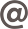FILTER

# Calculating

 Depth (mm) Weight (kg) Mould clearance volume (m³/pcs) Intra-concrete clearance m³/m² 225 9.30 0.116 0.143 325 11.40 0.158 0.195 425 13.40 0.19 0.240

Example:
Concrete volume used per unit m2 is calculated as follows:

Assume that the floor will be composed of ribs in 900 mm centre. Again, assume that the mould depth is 225 mm. The upper depth is 100 mm. Total depth is 325 mm.

Total full volume per m² = 1.0 x 1.0 x 0.325 = 0.325 m³ / m²
Clearance volume per m² = 0.143 m³ / m²
Concrete volume per m² = 0.325 - 0.143 = 0.182 m³ / m²

+90 212 671 0 681info@kasetkalip.com